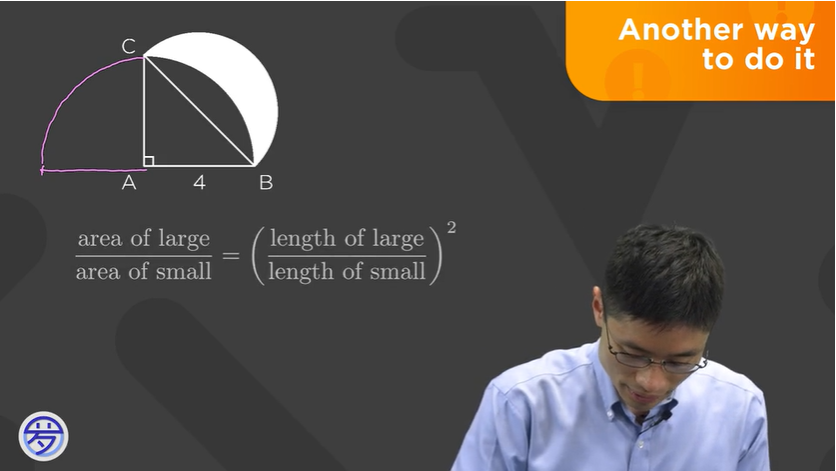# Why is the equation put together like this?

•• @victorioussheep Good question!

The equation comes from the fact that the ratio of the areas of two similar 2D figures is the square of the ratio of their lengths. (which is a really important thing to remember in geometry!)

We can prove the above statement for circles specifically, since that's the type of shape in the problem: say that circle O1 has radius x and circle O2 has radius kx (notice that the ratio of lengths here is k). Then, the area of circle O1 is (pi)x^2 and the area of circle O2 is (pi)(kx)^2 = k^2 * ((pi)x^2) = k^2 * (area of O1), so the ratio of areas is k^2.

We can also prove the statement for triangles: say triangle ABC ~ triangle DEF. Let the ratio of their side lengths be equal to k, so (AB)/(DE) = k or AB = k(DE). Now drop an altitude from C and let it hit AB at some point X. Also, drop an altitude from F so that it hits DE at Y. We know that (CX)/(FY) = k as well (this follows from the similarity)!

Now we can find [ABC] = bh/2 = (AB)(CX)/2 = k(DE) * k(FY)/2 = k^2 * (DE * FY/2).
Also, [DEF] = DE*FY/2, so [ABC] = k^2 * [DEF].

One way to see intuitively that the statement extends to polygons with more sides is to break each similar polygon into triangles in the same way (sort of like how you would to prove the polygon angle sum formula); the ratios of areas of each little triangle is k^2, as we found above, and you add them up to get the total areas.

In general, though, the intuition for the bolded statement is that lengths are 1D and areas are 2D, so when we go from a ratio of lengths to a ratio of areas, we need to square -- or scale by another factor. (As an extension, if you have similar 3D figures, the ratio of the volumes is the ratio of the side lengths, cubed!)

Hope that helped!• @audrey Thank you so much for answering my question! It helps really much!• @victorioussheep No problem!